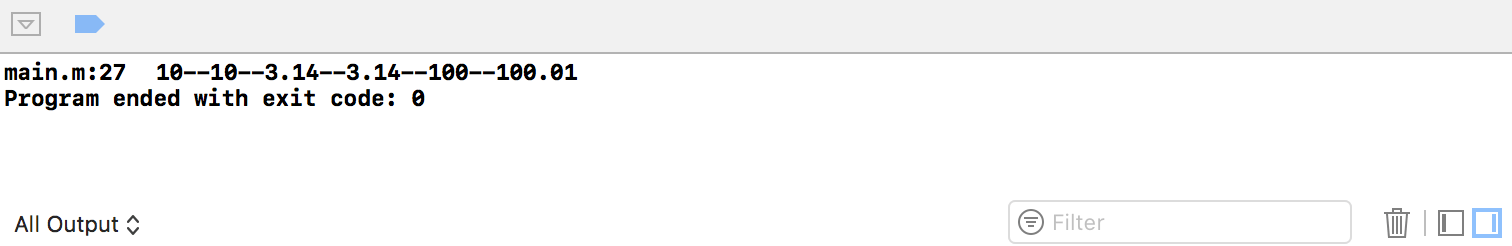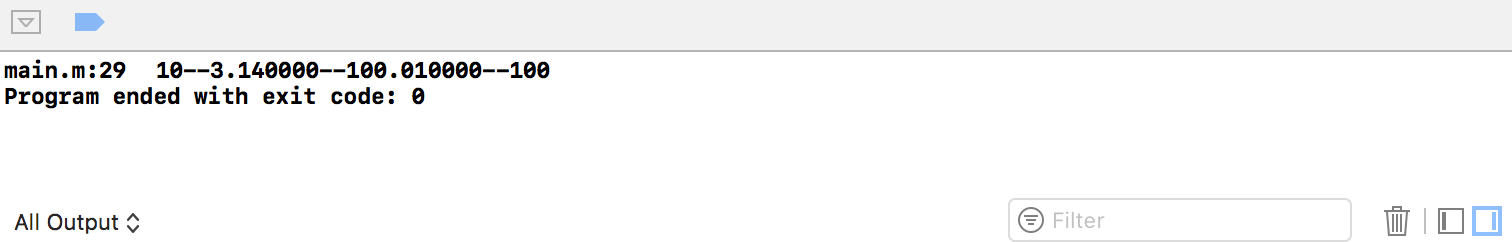# NSNumber对象创建

• 使用@符号进行快速封装；

• 调用NSNumber类提供的方法。通过调用NSNumber类中提供的方法，NSNumber类型的对象不仅仅可以转换int、float、double这些常规的基本数字类型，还可以包装char、BOOL，但并不常用。

``````+ (NSNumber *)numberWithInt:(int)value; //转换int
+ (NSNumber *)numberWithFloat:(float)value; //转换float
+ (NSNumber *)numberWithDouble:(double)value; //转换double
+ (NSNumber *)numberWithBool:(BOOL)value; //转换bool
+ (NSNumber *)numberWithInteger:(NSInteger)value; //转换NSInteger
``````

``````int main(int argc, const char * argv[]) {
@autoreleasepool {
//NSNumber对象创建
//快速创建
NSNumber * intNum1 = @10;
NSNumber * floatNum1 = @3.14;
//使用类方法创建
NSNumber * intNum2 = [NSNumber numberWithInt:10];
NSNumber * floatNum2 = [NSNumber numberWithFloat:3.14];
NSNumber * integerNum = [NSNumber numberWithInteger:100];
NSNumber * doubleNum = [NSNumber numberWithDouble:100.01];
NSLog(@"%@--%@--%@--%@--%@--%@", intNum1, intNum2,floatNum1,floatNum2,integerNum,doubleNum);
}
return 0;
}
``````# NSNumber对象与基本数据类型之间的转换

``````@property (readonly) int intValue; //NSNumber对象转成int
``````

``````int main(int argc, const char * argv[]) {
@autoreleasepool {
//使用类方法创建
NSNumber * intNum = [NSNumber numberWithInt:10];
NSNumber * floatNum = [NSNumber numberWithFloat:3.14];
NSNumber * integerNum = [NSNumber numberWithInteger:100];
NSNumber * doubleNum = [NSNumber numberWithDouble:100.01];

//转换成基本数据类型
int intBasic = [intNum intValue];
float floatBasic = [floatNum floatValue];
double doubleBasic = [doubleNum doubleValue];
NSInteger integerBasic = [integerNum integerValue];
NSLog(@"%d--%f--%f--%ld",intBasic,floatBasic, doubleBasic,(long)integerBasic);
}
return 0;
}
``````# 对比

• int ： 当使用int类型定义变量的时候，可以像写C程序一样去使用。当你不知道程序运行在哪种处理器架构时，你最好使用NSInteger，因为int在32位系统中也许只是int类型，而在64位系统中int可能变成long型。除非不得不使用int／long型，否则推荐使用NSInteger。

• NSInteger/NSUInteger是一种动态定义的类型，在不同的设备，不同的架构，有可能是int类型，有可能是long类型。NSUInteger是无符号的，即没有负数，NSInteger是有符号的。

• NSInteger是基础类型，NSNumber是一个类，如果需要在数组中存储一个数值，直接使用NSInteger是不行的，因为Objective-C的集合当中存储的数据必须是对象。

# 示例代码

https://github.com/99ios/4.5